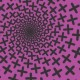# Hypocycloid Spiral

Actionscript:
1. x = stage.stageWidth / 2;
2. y = stage.stageHeight / 2;
3. // change n to alter number of spikes (cuspes)
4. var n:Number = 8;
5. var xp:Number = 0, yp:Number = 0, a:Number = 10, t:Number = 0;
6.
7. graphics.lineStyle(0, 0x000000);
9. function onLoop(evt:Event):void {
10.     for (var i:int = 0; i<10; i++){
11.       // unoptimized for simplicity and similarity to original equations from here:
12.           // http://mathworld.wolfram.com/Hypocycloid.html
13.       xp = a/n * ((n - 1) * Math.cos(t) + Math.cos(Math.abs((n - 1) * t)));
14.       yp = a/n * ((n - 1) * Math.sin(t) - Math.sin(Math.abs((n - 1) * t)))  ;
15.
16.       a *= 1.002;
17.        if (t == 0){
18.            graphics.moveTo(xp, yp);
19.        }else{
20.            graphics.lineTo(xp, yp);
21.        }
22.        t += .1;
23.     }
24. }

This code draws a Hypocycloid with a radius that increments - this results in a spiral formation.

I got the math from mathworld... here.

1.rauri
Posted April 20, 2009 at 1:39 am | Permalink

Ok, you’ve got the web, now where’s the spider?

2.Zevan
Posted April 20, 2009 at 7:56 am | Permalink

yeah, at first i didn’t realize that it looked like a spider web, needs some weights, some imperfection and some lines coming from the center going outward….